### Home > CC1 > Chapter 8 > Lesson 8.1.2 > Problem8-22

8-22.
1. Use <, >, or = to compare the number pairs below. Homework Help ✎

1. 0.183 ____ 0.18

2. −13 ____ − 17

3. 0.125 ____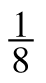4. −6 ____ −4

5. 72%____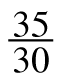6. −0.25____−0.05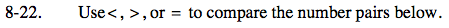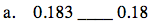Write both numbers to the thousandths place, then compare.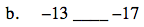It may help to place the numbers on a number line, like the one below.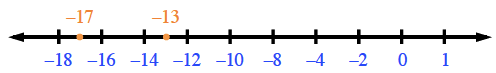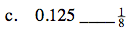Convert the fraction to a decimal, then compare.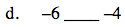See part (b).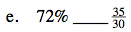Examine the fraction; is it greater than or less than 1?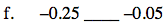See part (b).